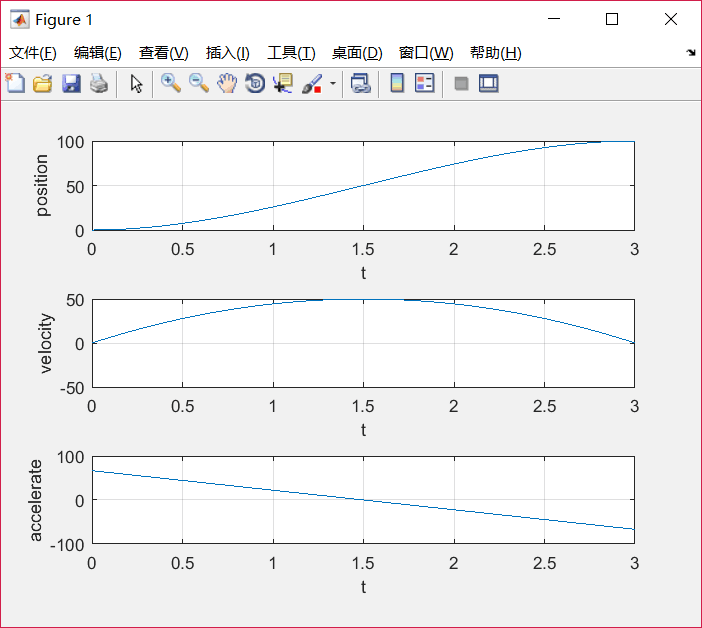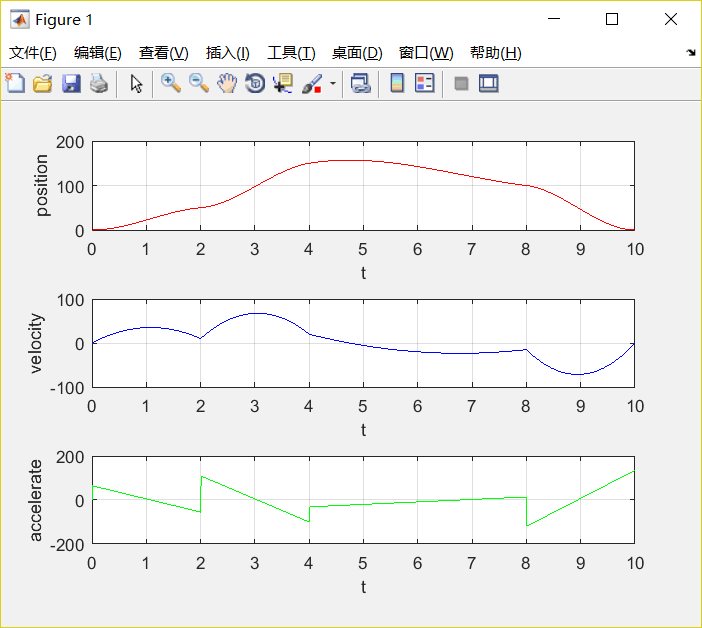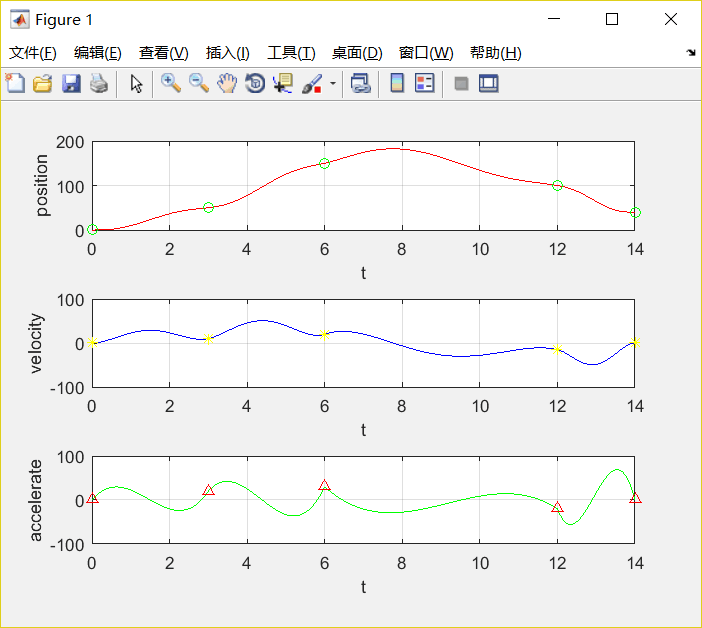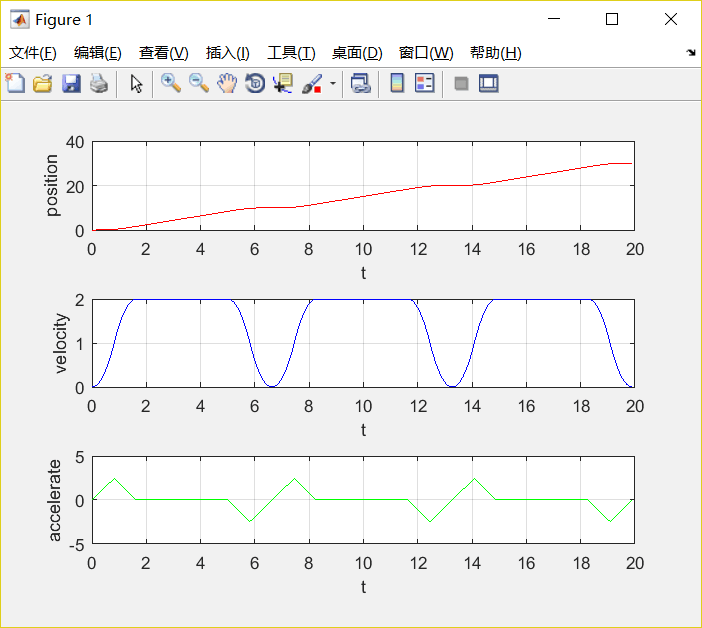• 工业机器人的轨迹规划是指综合考虑作业需求和机器人性能，在笛卡尔空间或关节空间内得出指导机器人末端执行器运动的轨迹。阐述了工业机器人轨迹规划的概念及其分类，就各个领域的轨迹规划算法进行了全面综述，包括...
• 运动规划指的是运动插补，在起始点和终止点之间插入中间点序列，实现沿着轨迹的平稳运动。运动控制包含路径规划(path planning)和轨迹规划，路径规划规划位置，在起终点之间经过路径点，轨迹规划规划时间，将...
1.轨迹规划的定义
轨迹规划(trajectory planning)是运动规划(motion planning)研究的主要内容。运动规划指的是运动插补，在起始点和终止点之间插入中间点序列，实现沿着轨迹的平稳运动。运动控制包含路径规划(path planning)和轨迹规划，路径规划是规划位置，在起终点之间经过的路径点，轨迹规划是规划时间，将路径点与时间相对应。
对于我们的六轴机器人而言轨迹规划可以分为：关节空间轨迹规划和笛卡尔空间轨迹规划。关节空间轨迹规划是把机器人的关节变量变换成跟时间的函数，然后对角速度和角加速度进行约束。笛卡尔空间轨迹规划是把机器人末端在笛卡尔空间的位移、速度和加速度变换成跟时间的函数关系。
2.数学基础
三次多项式插值（适用于起点和终点速度为零的情况，约束关节在起点和终点的角度值，规定轨迹两端点位置角速度为定值）。
设关节角满足下式
⎧⎩⎨⎪⎪⎪⎪θ(t)=a0+a1t+a2t2+a3t3θ˙(t)=a1+2a2t+3a3t2θ¨(t)=2a2+6a3t$\begin{array}{r}\left\{\begin{array}{l}\theta \left(t\right)={a}_{0}+{a}_{1}t+{a}_{2}{t}^{2}+{a}_{3}{t}^{3}\\ \stackrel{˙}{\theta }\left(t\right)={a}_{1}+2{a}_{2}t+3{a}_{3}{t}^{2}\\ \stackrel{¨}{\theta }\left(t\right)=2{a}_{2}+6{a}_{3}t\end{array}\end{array}$\begin{eqnarray*}
\left\{ \begin{array}{l}
\theta(t)=a_0+a_1t+a_2t^2+a_3t^3\\
\dot{\theta}(t)=a_1+2a_2t+3a_3t^2\\
\ddot{\theta}(t)=2a_2+6a_3t
\end{array}\right.
\end{eqnarray*}
将相邻两个点看作一小段轨迹的起点和终点分别用θ0${\theta }_{0}$$\theta_0$和θf${\theta }_{f}$$\theta_f$表示，约束起始速度为v0${v}_{0}$$v_0$，终止速度为vf${v}_{f}$$v_f$
⎧⎩⎨⎪⎪⎪⎪⎪⎪⎪⎪θ(t0)=θ0θ(tf)=θfθ˙(t0)=v0θ˙(tf)=vf$\begin{array}{r}\left\{\begin{array}{l}\theta \left({t}_{0}\right)={\theta }_{0}\\ \theta \left({t}_{f}\right)={\theta }_{f}\\ \stackrel{˙}{\theta }\left({t}_{0}\right)={v}_{0}\\ \stackrel{˙}{\theta }\left({t}_{f}\right)={v}_{f}\end{array}\end{array}$\begin{eqnarray*}
\left\{ \begin{array}{l}
\theta(t_0)=\theta_0\\
\theta(t_f)=\theta_f\\
\dot{\theta}(t_0)=v_0\\
\dot{\theta}(t_f)=v_f\\
\end{array}\right.
\end{eqnarray*}
将约束条件代入函数，可以求得系数（为简便计算，设t0=0${t}_{0}=0$$t_0=0$）
⎧⎩⎨⎪⎪⎪⎪⎪⎪⎪⎪⎪⎪⎪⎪a0=θ0a1=v0a2=3t2f(θf−θ0)−1tf(2v0+vf)a3=2t3f(θ0−θf)+1t2f(v0+vf)$\begin{array}{r}\left\{\begin{array}{l}{a}_{0}={\theta }_{0}\\ {a}_{1}={v}_{0}\\ {a}_{2}=\frac{3}{{t}_{f}^{2}}\left({\theta }_{f}-{\theta }_{0}\right)-\frac{1}{{t}_{f}}\left(2{v}_{0}+{v}_{f}\right)\\ {a}_{3}=\frac{2}{{t}_{f}^{3}}\left({\theta }_{0}-{\theta }_{f}\right)+\frac{1}{{t}_{f}^{2}}\left({v}_{0}+{v}_{f}\right)\end{array}\end{array}$\begin{eqnarray*}
\left\{ \begin{array}{l}
a_0=\theta_0\\
a_1=v_0\\
a_2=\frac{3}{t_f^2}(\theta_f-\theta_0)-\frac{1}{t_f}(2v_0+v_f)\\
a_3=\frac{2}{t_f^3}(\theta_0-\theta_f)+\frac{1}{t_f^2}(v_0+v_f)\\
\end{array}\right.
\end{eqnarray*}
三次多项式规划轨迹如下
⎧⎩⎨⎪⎪⎪⎪⎪⎪⎪⎪⎪⎪⎪⎪⎪⎪⎪⎪⎪⎪θ(t)=θ0+v0t+[3t2f(θf−θ0)−1tf(2v0+vf)]t2+[2t3f(θ0−θf)+1t2f(v0+vf)]t3θ˙(t)=v0+2[3t2f(θf−θ0)−1tf(2v0+vf)]t+3[2t3f(θ0−θf)+1t2f(v0+vf)]t2θ¨(t)=2[3t2f(θf−θ0)−1tf(2v0+vf)]+6[2t3f(θ0−θf)+1t2f(v0+vf)]t$\begin{array}{r}\left\{\begin{array}{l}\theta \left(t\right)={\theta }_{0}+{v}_{0}t+\left[\frac{3}{{t}_{f}^{2}}\left({\theta }_{f}-{\theta }_{0}\right)-\frac{1}{{t}_{f}}\left(2{v}_{0}+{v}_{f}\right)\right]{t}^{2}+\left[\frac{2}{{t}_{f}^{3}}\left({\theta }_{0}-{\theta }_{f}\right)+\frac{1}{{t}_{f}^{2}}\left({v}_{0}+{v}_{f}\right)\right]{t}^{3}\\ \stackrel{˙}{\theta }\left(t\right)={v}_{0}+2\left[\frac{3}{{t}_{f}^{2}}\left({\theta }_{f}-{\theta }_{0}\right)-\frac{1}{{t}_{f}}\left(2{v}_{0}+{v}_{f}\right)\right]t+3\left[\frac{2}{{t}_{f}^{3}}\left({\theta }_{0}-{\theta }_{f}\right)+\frac{1}{{t}_{f}^{2}}\left({v}_{0}+{v}_{f}\right)\right]{t}^{2}\\ \stackrel{¨}{\theta }\left(t\right)=2\left[\frac{3}{{t}_{f}^{2}}\left({\theta }_{f}-{\theta }_{0}\right)-\frac{1}{{t}_{f}}\left(2{v}_{0}+{v}_{f}\right)\right]+6\left[\frac{2}{{t}_{f}^{3}}\left({\theta }_{0}-{\theta }_{f}\right)+\frac{1}{{t}_{f}^{2}}\left({v}_{0}+{v}_{f}\right)\right]t\end{array}\end{array}$\begin{eqnarray*}
\left\{ \begin{array}{l}
\theta(t)=\theta_0+v_0t+[\frac{3}{t_f^2}(\theta_f-\theta_0)-\frac{1}{t_f}(2v_0+v_f)]t^2+[\frac{2}{t_f^3}(\theta_0-\theta_f)+\frac{1}{t_f^2}(v_0+v_f)]t^3\\
\dot{\theta}(t)=v_0+2[\frac{3}{t_f^2}(\theta_f-\theta_0)-\frac{1}{t_f}(2v_0+v_f)]t+3[\frac{2}{t_f^3}(\theta_0-\theta_f)+\frac{1}{t_f^2}(v_0+v_f)]t^2\\
\ddot{\theta}(t)=2[\frac{3}{t_f^2}(\theta_f-\theta_0)-\frac{1}{t_f}(2v_0+v_f)]+6[\frac{2}{t_f^3}(\theta_0-\theta_f)+\frac{1}{t_f^2}(v_0+v_f)]t
\end{array}\right.
\end{eqnarray*}
当速度为零时适用于一段轨迹的起终点，不为零时适用于一段轨迹的经过点。
3.matlab代码实现

序号
位置
速度
时间

1
0
0
0

2
100
0
3

clear;
clc;
q0=0;
q1=100; %指定起止位置
t0=0;
t1=3;%指定起止时间
v0=0;
v1=0;%指定起止速度
a0=q0;
a1=v0;
a2=(3/(t1)^2)*(q1-q0)-(1/t1)*(2*v0+v1);
a3=(2/(t1)^3)*(q0-q1)+(1/t1^2)*(v0+v1);%计算三次多项式系数
t=t0:0.01:t1;
q=a0+a1*t+a2*t.^2+a3*t.^3;%三次多项式插值的位置
v=a1+2*a2*t+3*a3*t.^2;%三次多项式插值的速度
a=2*a2+6*a3*t;%三次多项式插值的加速度
subplot(3,1,1),plot(t,q),xlabel('t'),ylabel('position');grid on;
subplot(3,1,2),plot(t,v),xlabel('t'),ylabel('velocity');grid on;
subplot(3,1,3),plot(t,a),xlabel('t'),ylabel('accelerate');grid on;

下图为插值结果4.含经过点的插值

序号
位置
速度
时间

1
0
0
0

2
50
10
3

3
150
20
6

4
100
-15
12

5
0
0
14

clear;
clc;
q_array=[0,50,150,100,0];%指定起止位置
t_array=[0,2,4,8,10];%指定起止时间
v_array=[0,10,20,-15,0];%指定起止速度
t=[t_array(1)];q=[q_array(1)];v=[v_array(1)];a=;%初始状态
for i=1:1:length(q_array)-1;%每一段规划的时间
a0=q_array(i);
a1=v_array(i);
a2=(3/(t_array(i+1)-t_array(i))^2)*(q_array(i+1)-q_array(i))-(1/(t_array(i+1)-t_array(i)))*(2*v_array(i)+v_array(i+1));
a3=(2/(t_array(i+1)-t_array(i))^3)*(q_array(i)-q_array(i+1))+(1/(t_array(i+1)-t_array(i))^2)*(v_array(i)+v_array(i+1));%计算三次多项式系数
ti=t_array(i)+0.001:0.001:t_array(i+1);
qi=a0+a1*(ti-t_array(i))+a2*(ti-t_array(i)).^2+a3*(ti-t_array(i)).^3;
vi=a1+2*a2*(ti-t_array(i))+3*a3*(ti-t_array(i)).^2;
ai=2*a2+6*a3*(ti-t_array(i));
t=[t,ti];q=[q,qi];v=[v,vi];a=[a,ai];
end
subplot(3,1,1),plot(t,q,'r'),xlabel('t'),ylabel('position');grid on;
subplot(3,1,2),plot(t,v,'b'),xlabel('t'),ylabel('velocity');grid on;
subplot(3,1,3),plot(t,a,'g'),xlabel('t'),ylabel('accelerate');grid on;PS:这种三次多项式插值，只要给定离散点位置、速度和时间，就能插补出一段连续平滑的曲线。但是角加速度并不连续，下次为大家介绍高阶多项式插值则可以解决这个问题。
展开全文插值算法 三次多项式插值
• 此仓库将托管我的研究代码，用于研究不同机器人系统的轨迹优化。 我的最初计划实施论文“使用隐式硬接触进行轨迹优化”，并研究本文提到的三个工具： 杂技机器人 第一个系统：直接搭配的acrobot 首先需要运行“〜/...
• 运动规划指的是运动插补，在起始点和终止点之间插入中间点序列，实现沿着轨迹的平稳运动。运动控制包含路径规划(path planning)和轨迹规划，路径规划规划位置，在起终点之间经过路径点，轨迹规划规划时间，将...
1.轨迹规划的定义
轨迹规划(trajectory planning)是运动规划(motion planning)研究的主要内容。运动规划指的是运动插补，在起始点和终止点之间插入中间点序列，实现沿着轨迹的平稳运动。运动控制包含路径规划(path planning)和轨迹规划，路径规划是规划位置，在起终点之间经过的路径点，轨迹规划是规划时间，将路径点与时间相对应。
对于我们的六轴机器人而言轨迹规划可以分为：关节空间轨迹规划和笛卡尔空间轨迹规划。关节空间轨迹规划是把机器人的关节变量变换成跟时间的函数，然后对角速度和角加速度进行约束。笛卡尔空间轨迹规划是把机器人末端在笛卡尔空间的位移、速度和加速度变换成跟时间的函数关系。
五次多项式插值能够解决三次多项式插值的角速度变化不平滑且加速度存在跳变的情况。
2.数学基础
五次多项式插值，角位移、角速度和角加速度的函数表达式为：
⎧⎩⎨⎪⎪⎪⎪θ(t)=a0+a1t+a2t2+a3t3+a4t4+a5t5θ˙(t)=a1+2a2t+3a3t2+4a4t3+5a5t4θ¨(t)=2a2+6a3t+12a4t2+20a5t3$\begin{array}{r}\left\{\begin{array}{l}\theta \left(t\right)={a}_{0}+{a}_{1}t+{a}_{2}{t}^{2}+{a}_{3}{t}^{3}+{a}_{4}{t}^{4}+{a}_{5}{t}^{5}\\ \stackrel{˙}{\theta }\left(t\right)={a}_{1}+2{a}_{2}t+3{a}_{3}{t}^{2}+4{a}_{4}{t}^{3}+5{a}_{5}{t}^{4}\\ \stackrel{¨}{\theta }\left(t\right)=2{a}_{2}+6{a}_{3}t+12{a}_{4}{t}^{2}+20{a}_{5}{t}^{3}\end{array}\end{array}$\begin{eqnarray*}
\left\{ \begin{array}{l}
\theta(t)=a_0+a_1t+a_2t^2+a_3t^3+a_4t^4+a_5t^5\\
\dot{\theta}(t)=a_1+2a_2t+3a_3t^2+4a_4t^3+5a_5t^4\\
\ddot{\theta}(t)=2a_2+6a_3t+12a_4t^2+20a_5t^3
\end{array}\right.
\end{eqnarray*}
约束条件如下，相对三次多项式插值，增加了对起止点角速度的约束（为简便计算设t0${t}_{0}$$t_0$为0）
⎧⎩⎨⎪⎪⎪⎪⎪⎪⎪⎪⎪⎪⎪⎪⎪⎪⎪⎪⎪⎪⎪⎪⎪⎪⎪⎪θ(t0)=θ0=a0θ(tf)=θf=a0+a1tf+a2t2f+a3t3f+a4t4f+a5t5fθ˙(t0)=θ˙0=a1θ˙(tf)=θ˙f=a1+2a2tf+3a3t2f+4a4t3f+5a5t4fθ¨(t0)=θ¨0=2a2θ¨(tf)=θ¨f=2a2+6a3tf+12a4t2f+20a5t3f$\begin{array}{r}\left\{\begin{array}{l}\theta \left({t}_{0}\right)={\theta }_{0}={a}_{0}\\ \theta \left({t}_{f}\right)={\theta }_{f}={a}_{0}+{a}_{1}{t}_{f}+{a}_{2}{t}_{f}^{2}+{a}_{3}{t}_{f}^{3}+{a}_{4}{t}_{f}^{4}+{a}_{5}{t}_{f}^{5}\\ \stackrel{˙}{\theta }\left({t}_{0}\right)={\stackrel{˙}{\theta }}_{0}={a}_{1}\\ \stackrel{˙}{\theta }\left({t}_{f}\right)={\stackrel{˙}{\theta }}_{f}={a}_{1}+2{a}_{2}{t}_{f}+3{a}_{3}{t}_{f}^{2}+4{a}_{4}{t}_{f}^{3}+5{a}_{5}{t}_{f}^{4}\\ \stackrel{¨}{\theta }\left({t}_{0}\right)={\stackrel{¨}{\theta }}_{0}=2{a}_{2}\\ \stackrel{¨}{\theta }\left({t}_{f}\right)={\stackrel{¨}{\theta }}_{f}=2{a}_{2}+6{a}_{3}{t}_{f}+12{a}_{4}{t}_{f}^{2}+20{a}_{5}{t}_{f}^{3}\end{array}\end{array}$\begin{eqnarray*}
\left\{ \begin{array}{l}
\theta(t_0)=\theta_0=a_0\\
\theta(t_f)=\theta_f=a_0+a_1t_f+a_2t_f^2+a_3t_f^3+a_4t_f^4+a_5t_f^5\\
\dot{\theta}(t_0)=\dot{\theta}_0=a_1\\
\dot{\theta}(t_f)=\dot{\theta}_f=a_1+2a_2t_f+3a_3t_f^2+4a_4t_f^3+5a_5t_f^4\\
\ddot{\theta}(t_0)=\ddot{\theta}_0=2a_2\\
\ddot{\theta}(t_f)=\ddot{\theta}_f=2a_2+6a_3t_f+12a_4t_f^2+20a_5t_f^3
\end{array}\right.
\end{eqnarray*}
求解得
⎧⎩⎨⎪⎪⎪⎪⎪⎪⎪⎪⎪⎪⎪⎪⎪⎪⎪⎪⎪⎪⎪⎪⎪⎪⎪⎪⎪⎪⎪⎪⎪⎪⎪⎪⎪⎪a0=θ0a1=θ˙0a2=θ¨02a3=20θf−20θ0−(8θ˙f+12θ˙0)tf−(3θ¨0−θ¨f)t2f2t3fa4=30θ0−30θf+(14θ˙f+16θ˙0)tf+(3θ¨0−2θ¨f)t2f2t4fa5=12θf−12θ0−(6θ˙f+6θ˙0)tf−(θ¨0−θ¨f)t2f2t5f$\begin{array}{r}\left\{\begin{array}{l}{a}_{0}={\theta }_{0}\\ {a}_{1}={\stackrel{˙}{\theta }}_{0}\\ {a}_{2}=\frac{{\stackrel{¨}{\theta }}_{0}}{2}\\ {a}_{3}=\frac{20{\theta }_{f}-20{\theta }_{0}-\left(8{\stackrel{˙}{\theta }}_{f}+12{\stackrel{˙}{\theta }}_{0}\right){t}_{f}-\left(3{\stackrel{¨}{\theta }}_{0}-{\stackrel{¨}{\theta }}_{f}\right){t}_{f}^{2}}{2{t}_{f}^{3}}\\ {a}_{4}=\frac{30{\theta }_{0}-30{\theta }_{f}+\left(14{\stackrel{˙}{\theta }}_{f}+16{\stackrel{˙}{\theta }}_{0}\right){t}_{f}+\left(3{\stackrel{¨}{\theta }}_{0}-2{\stackrel{¨}{\theta }}_{f}\right){t}_{f}^{2}}{2{t}_{f}^{4}}\\ {a}_{5}=\frac{12{\theta }_{f}-12{\theta }_{0}-\left(6{\stackrel{˙}{\theta }}_{f}+6{\stackrel{˙}{\theta }}_{0}\right){t}_{f}-\left({\stackrel{¨}{\theta }}_{0}-{\stackrel{¨}{\theta }}_{f}\right){t}_{f}^{2}}{2{t}_{f}^{5}}\end{array}\end{array}$\begin{eqnarray*}
\left\{ \begin{array}{l}
a_0=\theta_0\\
a_1=\dot{\theta}_0\\
a_2=\frac{\ddot{\theta}_0}{2}\\
a_3=\frac{20\theta_f-20\theta_0-(8\dot\theta_f+12\dot\theta_0)t_f-(3\ddot\theta_0-\ddot\theta_f)t_f^2}{2t_f^3}\\
a_4=\frac{30\theta_0-30\theta_f+(14\dot\theta_f+16\dot\theta_0)t_f+(3\ddot\theta_0-2\ddot\theta_f)t_f^2}{2t_f^4}\\
a_5=\frac{12\theta_f-12\theta_0-(6\dot\theta_f+6\dot\theta_0)t_f-(\ddot\theta_0-\ddot\theta_f)t_f^2}{2t_f^5}\\
\end{array}\right.
\end{eqnarray*}
3.matlab代码实现

序号
位移
速度
加速度
时间

1
0
0
0
0

2
50
10
20
3

3
150
20
30
6

4
100
-15
-20
12

5
0
0
0
14

clear;
clc;
q_array=[0,50,150,100,40];%指定起止位置
t_array=[0,3,6,12,14];%指定起止时间
v_array=[0,10,20,-15,0];%指定起止速度
a_array=[0,20,30,-20,0];%指定起止加速度
t=[t_array(1)];q=[q_array(1)];v=[v_array(1)];a=[a_array(1)];%初始状态
for i=1:1:length(q_array)-1;%每一段规划的时间
T=t_array(i+1)-t_array(i)
a0=q_array(i);
a1=v_array(i);
a2=a_array(i)/2;
a3=(20*q_array(i+1)-20*q_array(i)-(8*v_array(i+1)+12*v_array(i))*T-(3*a_array(i)-a_array(i+1))*T^2)/(2*T^3);
a4=(30*q_array(i)-30*q_array(i+1)+(14*v_array(i+1)+16*v_array(i))*T+(3*a_array(i)-2*a_array(i+1))*T^2)/(2*T^4);
a5=(12*q_array(i+1)-12*q_array(i)-(6*v_array(i+1)+6*v_array(i))*T-(a_array(i)-a_array(i+1))*T^2)/(2*T^5);%计算五次多项式系数
ti=t_array(i):0.001:t_array(i+1);
qi=a0+a1*(ti-t_array(i))+a2*(ti-t_array(i)).^2+a3*(ti-t_array(i)).^3+a4*(ti-t_array(i)).^4+a5*(ti-t_array(i)).^5;
vi=a1+2*a2*(ti-t_array(i))+3*a3*(ti-t_array(i)).^2+4*a4*(ti-t_array(i)).^3+5*a5*(ti-t_array(i)).^4;
ai=2*a2+6*a3*(ti-t_array(i))+12*a4*(ti-t_array(i)).^2+20*a5*(ti-t_array(i)).^3;
t=[t,ti(2:end)];q=[q,qi(2:end)];v=[v,vi(2:end)];a=[a,ai(2:end)];
end
subplot(3,1,1),plot(t,q,'r'),xlabel('t'),ylabel('position');hold on;plot(t_array,q_array,'o','color','g'),grid on;
subplot(3,1,2),plot(t,v,'b'),xlabel('t'),ylabel('velocity');hold on;plot(t_array,v_array,'*','color','y'),grid on;
subplot(3,1,3),plot(t,a,'g'),xlabel('t'),ylabel('accelerate');hold on;plot(t_array,a_array,'^','color','r'),grid on;PS:相对于三次多项式插值，加速度也是平滑的曲线，并没有出现跳变的情况。然而在机器人系统中，单纯的多项式规划有一个非常严重的问题：没有匀速段，无法根据期望速度提供匀速控制，而在大部分机器人应用中，对加工的速度控制都是有要求的。另一个问题就是，次数越高的多项式，加速过程越慢，整个运动过程中的平均速度越小，影响效率。下次将会为大家介绍能约束速度的三段s曲线加减速直线插值方式。
展开全文五次多项式插值
• 运动规划指的是运动插补，在起始点和终止点之间插入中间点序列，实现沿着轨迹的平稳运动。运动控制包含路径规划(path planning)和轨迹规划，路径规划规划位置，在起终点之间经过路径点，轨迹规划规划时间，将...
1.原理
五段s曲线相较于三段s曲线而言加速度也是连续变化的，能适用于平稳性要求更高的场合。分为加加速、加减速、匀速、减加速、减减速这五段。
设除匀速段以为，其余四段的时间相等都为Ta${T}_{a}$$T_a$，总时间为T$T$$T$，匀速段速度为vs${v}_{s}$$v_s$，四个变速段斜率大小都为A$A$$A$，整段轨迹的总位移L$L$$L$、加加速段位移L1${L}_{1}$$L_1$、加减速段位移L2${L}_{2}$$L_2$
⎧⎩⎨⎪⎪⎪⎪⎪⎪⎪⎪⎪⎪⎪⎪⎪⎪Ta=vsA−−√L1=16AT3aL2=56AT3aT=4Ta+L−2L1−2L2vs$\begin{array}{r}\left\{\begin{array}{l}{T}_{a}=\sqrt{\frac{{v}_{s}}{A}}\\ {L}_{1}=\frac{1}{6}A{T}_{a}^{3}\\ {L}_{2}=\frac{5}{6}A{T}_{a}^{3}\\ T=4{T}_{a}+\frac{L-2{L}_{1}-2{L}_{2}}{{v}_{s}}\end{array}\end{array}$\begin{eqnarray*}
\left\{ \begin{array}{l}
T_a=\sqrt{\frac{v_s}{A}}\\
L_1=\frac{1}{6}AT_a^3\\
L_2=\frac{5}{6}AT_a^3\\
T=4T_a+\frac{L-2L_1-2L_2}{v_s}
\end{array}\right.
\end{eqnarray*}
则加速度分段函数为
a=⎧⎩⎨⎪⎪⎪⎪⎪⎪⎪⎪⎪⎪⎪⎪At,(0≤t≤Ta)−A(t−2Ta),(Ta≤t≤2Ta)0,(2Ta≤t≤T−2Ta)−A[t−(T−2Ta)],(T−2Ta≤t≤T−Ta)A(t−T),(T−Ta≤t≤T)$\begin{array}{r}a=\left\{\begin{array}{l}At,\left(0\le t\le {T}_{a}\right)\\ -A\left(t-2{T}_{a}\right),\left({T}_{a}\le t\le 2{T}_{a}\right)\\ 0,\left(2{T}_{a}\le t\le T-2{T}_{a}\right)\\ -A\left[t-\left(T-2{T}_{a}\right)\right],\left(T-2{T}_{a}\le t\le T-{T}_{a}\right)\\ A\left(t-T\right),\left(T-{T}_{a}\le t\le T\right)\end{array}\end{array}$\begin{eqnarray*}
a= \left\{ \begin{array}{l}
At,(0\leq{t}\leq{T_a})\\
-A(t-2T_a),({T_a}\leq{t}\leq2{T_a})\\
0,({2T_a}\leq{t}\leq{T-2{T_a}})\\
-A[t-(T-2T_a)],(T-2{T_a}\leq{t}\leq{T-{T_a}})\\
A(t-T),(T-{T_a}\leq{t}\leq{T})
\end{array}\right.
\end{eqnarray*}
对加速度积分可得
v=⎧⎩⎨⎪⎪⎪⎪⎪⎪⎪⎪⎪⎪⎪⎪⎪⎪⎪⎪12At2,(0≤t≤Ta)−12A(t−2Ta)2+AT2a,(Ta≤t≤2Ta)vs,(2Ta≤t≤T−2Ta)−12A（t−T+2Ta)2+AT2a,(T−2Ta≤t≤T−Ta)12A(t−T)2,(T−Ta≤t≤T)$\begin{array}{r}v=\left\{\begin{array}{l}\frac{1}{2}A{t}^{2},\left(0\le t\le {T}_{a}\right)\\ -\frac{1}{2}A\left(t-2{T}_{a}{\right)}^{2}+A{T}_{a}^{2},\left({T}_{a}\le t\le 2{T}_{a}\right)\\ {v}_{s},\left(2{T}_{a}\le t\le T-2{T}_{a}\right)\\ -\frac{1}{2}A（t-T+2{T}_{a}{\right)}^{2}+A{T}_{a}^{2},\left(T-2{T}_{a}\le t\le T-{T}_{a}\right)\\ \frac{1}{2}A\left(t-T{\right)}^{2},\left(T-{T}_{a}\le t\le T\right)\end{array}\end{array}$\begin{eqnarray*}
v=\left\{ \begin{array}{l}
\frac{1}{2}At^2,(0\leq{t}\leq{T_a})\\
-\frac{1}{2}A(t-2T_a)^2+AT_a^2,({T_a}\leq{t}\leq2{T_a})\\
v_s,({2T_a}\leq{t}\leq{T-2{T_a}})\\
-\frac{1}{2}A（t-T+2T_a)^2+AT_a^2,(T-2{T_a}\leq{t}\leq{T-{T_a}})\\
\frac{1}{2}A(t-T)^2,(T-{T_a}\leq{t}\leq{T})
\end{array}\right.
\end{eqnarray*}
对速度积分可得到位移s的分段函数
s=⎧⎩⎨⎪⎪⎪⎪⎪⎪⎪⎪⎪⎪⎪⎪⎪⎪⎪⎪16At3,(0≤t≤Ta)−16A(t−2Ta)3+AT2at−AT3a,(Ta≤t≤2Ta)AT2at−AT3a,(2Ta≤t≤T−2Ta)−16A(t−T+2Ta)3+AT2at−AT2a,(T−2Ta≤t≤T−Ta)16A(t−T)3−2AT3a+AT2bT,(T−Ta≤t≤T)$\begin{array}{r}s=\left\{\begin{array}{l}\frac{1}{6}A{t}^{3},\left(0\le t\le {T}_{a}\right)\\ -\frac{1}{6}A\left(t-2{T}_{a}{\right)}^{3}+A{T}_{a}^{2}t-A{T}_{a}^{3},\left({T}_{a}\le t\le 2{T}_{a}\right)\\ A{T}_{a}^{2}t-A{T}_{a}^{3},\left(2{T}_{a}\le t\le T-2{T}_{a}\right)\\ -\frac{1}{6}A\left(t-T+2{T}_{a}{\right)}^{3}+A{T}_{a}^{2}t-A{T}_{a}^{2},\left(T-2{T}_{a}\le t\le T-{T}_{a}\right)\\ \frac{1}{6}A\left(t-T{\right)}^{3}-2A{T}_{a}^{3}+A{T}_{b}^{2}T,\left(T-{T}_{a}\le t\le T\right)\end{array}\end{array}$\begin{eqnarray*}
s= \left\{ \begin{array}{l}
\frac{1}{6}At^3,(0\leq{t}\leq{T_a})\\
-\frac{1}{6}A(t-2T_a)^3+AT_a^2t-AT_a^3,({T_a}\leq{t}\leq2{T_a})\\
AT_a^2t-AT_a^3,({2T_a}\leq{t}\leq{T-2{T_a}})\\
-\frac{1}{6}A(t-T+2T_a)^3+AT_a^2t-AT_a^2,(T-2{T_a}\leq{t}\leq{T-{T_a}})\\
\frac{1}{6}A(t-T)^3-2AT_a^3+AT_b^2T,(T-{T_a}\leq{t}\leq{T})
\end{array}\right.
\end{eqnarray*}
3.matlab代码实现
指定位置、速度、斜率

clc;
clear;
%初始条件
x_arry=[0,10,20,30];
v_arry=[2,2,2];
A_arry=[3,3,3];
weiyi=[x_arry(1)];sudu=;shijian=;timeall=0;jiasudu=
for i=1:1:length(x_arry)-1;
%清空
a=[];v=[];s=[];
%计算加减速段的时间和位移
L=x_arry(i+1)-x_arry(i);
A=A_arry(i);
vs=v_arry(i);
Ta=sqrt(vs/A);
L1=A*(Ta^3)/6;
L2=A*(Ta^3)*(5/6);
%计算整段轨迹的总位移
T=4*Ta+(L-2*L1-2*L2)/vs;
for t=0:0.001:T
if t<=Ta;%加加速度阶段
ad=A*t;
vd=0.5*A*t^2;
sd=(1/6)*A*t^3;
a=[a,ad];v=[v,vd];s=[s,sd];
elseif t>Ta && t<=2*Ta;%加减速阶段
ad=-A*(t-2*Ta);
vd=-0.5*A*(t-2*Ta)^2+A*Ta^2;
sd=-(1/6)*A*(t-2*Ta)^3+A*Ta^2*t-A*Ta^3;
a=[a,ad];v=[v,vd];s=[s,sd];
elseif t>2*Ta && t<=T-2*Ta;%匀速阶段
ad=0;
vd=vs;
sd=A*Ta^2*t-A*Ta^3;
a=[a,ad];v=[v,vd];s=[s,sd];
elseif t>T-2*Ta && t<=T-Ta;%减加度阶段
ad=-A*(t-(T-2*Ta));
vd=-0.5*A*(t-T+2*Ta)^2+A*Ta^2;
sd=-(1/6)*A*(t-T+2*Ta)^3+A*Ta^2*t-A*Ta^3;
a=[a,ad];v=[v,vd];s=[s,sd];
elseif t>T-Ta && t<=T;%减减阶段
ad=A*(t-T);
vd=0.5*A*(t-T)^2;
sd=(1/6)*A*(t-T)^3-2*A*Ta^3+A*Ta^2*T;
a=[a,ad];v=[v,vd];s=[s,sd];
end
end
%时间
time=[timeall:0.001:timeall+T];
timeall=timeall+T;
%连接每一段轨迹
weiyi=[weiyi,s(2:end)+x_arry(i)];
sudu=[sudu,v(2:end)];
jiasudu=[jiasudu,a(2:end)];
shijian=[shijian,time(2:end)];
end
subplot(3,1,1),plot(shijian,weiyi,'r');xlabel('t'),ylabel('position');grid on;
subplot(3,1,2),plot(shijian,sudu,'b');xlabel('t'),ylabel('velocity');grid on;
subplot(3,1,3),plot(shijian,jiasudu,'g');xlabel('t'),ylabel('accelerate');grid on;

结果如下展开全文matlab
• 所谓轨迹是指操作臂在运动过程中位移、速度和加速度。而轨迹规划是根据作业任务要求，计算出预期运动轨迹。通常将操作臂运动看作是工具坐标系相对于工作坐标系运动。对于点位作业，需要描述它起始状态...
所谓轨迹、是指操作臂在运动过程中的位移、速度和加速度。而轨迹规划是根据作业任务的要求，计算出预期的运动轨迹。通常将操作臂的运动看作是工具坐标系相对于工作坐标系的运动。对于点位作业，需要描述它的起始状态和目标状态，对于曲面加工，不仅要规定操作臂的起始点和终止点，而且要指明两点之间的若干中间点（称路径点）、必须沿特定的路径运动（路径约束）。这类称为连续路径运动或轮廓运动。
在规划机器人的运动时，还需要弄清楚在其路径上是否存在障碍物（障碍约束）。路径约束和障碍约束的组合将机器人的规划与控制方式划分为4类。PP95
轨迹规划器可看成是黑箱，其输入包括路径的“设定”和“约束”，输出是操作臂末端手部的“位姿序列”，表示手部在各个离散时刻的中间形位。操作臂最常用的轨迹规划方法有两种：第一种要求用户对于选定的轨迹结点（插值点）上的位姿、速度和加速度给出一组显式约束（例如连续性和光滑程度等），轨迹规划器从一类函数（例如n次多项式）中选取参数化轨迹，对结点进行插值，并满足约束条件。第二种方法要求用户给出运动路径的解析式；如直角坐标空间中的直线路径，轨迹规划器在关节空间或直角坐标空间中确定一条轨迹来逼近预定的路径。第一种方法中，约束的设定和轨迹规划均在关节空间进行。由于对操作臂手部（直角坐标形位）没有施加任何约束，用户很难弄清手部的实际路径，因此可能会发生与障碍物相碰。第二种方法的路径约束是在直角坐标空间中给定的，而关节驱动器是在关节空间中受控的。因此，为了得到与给定路径十分接近的轨迹，首先不许采用某种函数逼近的方法将直角坐标路径约束转化为关节坐标路径约束，然后确定满足关节路径约束的参数化路径。
轨迹规划既可在关节空间也可在直角空间中进行，但是做规划的轨迹函数都必须连续和平滑，使得操作臂的运动平稳。在关节空间进行规划时，是将关节变量表示成为时间的函数，并规划它的一阶和二阶时间导数；在直角空间进行规划是指将手部位姿、速度和加速度表示为时间的函数。而相应的关节位移、速度和加速度由手部的信息导出。通常通过运动学反解得出关节位移，用逆雅克比求出关节速度，用逆雅克比及其导数求解关节加速度。
用户根据作业给出各个路径结点后，规划器的任务包含：解变换方程、进行运动学反解和插值运算等；在关节空间进行规划时，大量工作是对关节边路的插值运算。
关节轨迹的插值：为了求得在关节空间形成所要求的轨迹，首先运用运动学反解将路径点转换成关节矢量角度值，然后对每个关节拟合一个光滑函数，使之从起始点开始，依次通过所有路径点，最后到达目标点。对于每一段路径，各个关节运动时间均相同，这样保证所有关节同时到达路径点和终止点，从而得到工具坐标系应有的位置和姿态。但是，尽管每个关节在同一段路径中的运动时间相同，各个关节函数之间却是相互独立的。
总之，关节空间法是以关节角度的函数来描述机器人的轨迹的，关节空间法不必在直角坐标系中描述两个路径点之间的路径形状，计算简单，容易。再者，由于关节空间与直角坐标空间之间不是连续的对应关系，因而不回发生机构的奇异性问题。
在关节空间中进行轨迹规划，需要给定机器人在起始点、终止点手臂的形位。对关节进行插值时，应满足一系列约束条件。在满足所有约束条件下，可以选取不同类型的关节插值函数，生成不同的轨迹。插值方法有：
1、三次多项式插值；2、过路径点的三次多项式插值；3、高阶多项式插值；4、用抛物线过渡的线性插值；5、过路径点的用抛物线过渡的线性插值。
笛卡尔空间规划法：
一、物体对象的描述
相对于固接坐标系，物体上任一点用相应的位置矢量表示，任一方向用方向余弦表示，给出物体的几何图形及固接坐标系后，只要规定固接坐标系的位姿，便可重构该物体。
二、作业的描述
在这种轨迹规划系统中，作业是用操作臂终端抓手位姿的笛卡尔坐标结点序列规定的，因此，结点是指表示抓手位姿的齐次变换矩阵。相应的关节变量可用运动学反解程序计算。
三、两个结点之间的“直线”运动
操作臂在完成作业时，抓手的位姿可以用一系列结点P来表示。因此，在直角坐标空间中进行轨迹规划的首要问题是由两结点pi和pi+1所定义的路径起点和终点之间，如何生成一系列中间点。两结点间最简单的路径是在空间的一个直线移动和绕某轴的转动。若运动时间给定之后，则可产生一个使线速度和角速度受控的运动。
四、两段路径之间的过渡
为了避免两段路径衔接点处速度不连续，当由一段轨迹过渡到下一段轨迹时，需要加速或减速。
五、运动学反解的有关问题
主要是笛卡尔路径上解的存在性（路径点都在工作空间之内与否）、唯一性和奇异性。
1、  第一类问题：中间点在工作空间之外。在关节空间中进行规划不会出现这类问题。
2、  第二类问题：在奇异点附近关节速度激增。想PUMA这类机器人具有两种奇异点：工作空间边界奇异点和工作空间内部的奇异点。在处于奇异位姿时，与操作速度（笛卡尔空间速度）相对应的关节速度可能不存在（无限大）。可以想象，当沿笛卡尔空间的直线路径运动到奇异点附近时，某些关节速度将会趋于无限大。实际上，所容许的关机速度是有限的，因而会导致操作臂偏离预期轨迹。
3、  第三类问题：起始点和目标点有多重解。问题在于起始点与目标点若不用同一个反解，这时关节变量的约束和障碍约束便会产生问题。
正因为笛卡尔空间轨迹存在这些问题，现有的多数工业机器人的控制系统具有关节空间和笛卡尔空间的轨迹生成方法。用户通常使用关节空间法，只是在必要时，才采用笛卡尔空间方法。
展开全文任务 工具
• 轨迹的作用使机器人系统通过计算机计算出来的轨迹点形成的轨迹进行规划运动，从而避免人为对机器人运动的所有状态进行定义，减少人机交互的过程。轨迹点以某种速率被计算的，称为路径更新速率。 路径要解决的...
• 因此近几年来，欠驱动机器人的运动控制问题引起国内外广泛的研究兴趣。同时，随着集成芯片技术的飞速发展，人们对欠驱动机器人控制的实时性和精度提出了越来越高的要求。 　本文设计了一种基于DSP的机器人控制系统...
• 智能机器人是指能够自主完成特定任务的机器人，而对环境感知和运动规 划的研究则有助于提高机器人的自主性，使其更加智能化。环境感知主要是通 过各类视觉传感器采集点云或图像数据，并由计算机进行分析处理后，使...
•  步态：是指机器人的每条腿按一定的顺序和轨迹的运动过程，正是因为这一运动过程实现了机器人的步行运动。  步态周期：步态周期是指多足机器人完成一个步态所需的时间，也就是所有腿轮番完成一次“提起-摆动-放下...
• 移动机器人路径规划问题 移动机器人运动轨迹规划是移动机器人导航技术的核心技术之一。我认为移动机器人的导航技术无疑是在解决三个问题：①我现在在何处？...移动机器人的运动路径规划是指机器人从起始点到目标算法
• 轨迹是指每个自由度的位置、速度、加速度的时间历程。这个问题包括如何通过人机交互制定通过空间的一条轨迹或路径。 在这里我们不要求用户写出复杂的时间和空间的函数来指定机器人任务，反而要使他们简单描述机器人...
• 根据控制律，选取图中所示的多个初始位置（棕色圆点所述，箭头是指机器人的正向），进行仿真实验， 画出仿真得到的轨迹
• CP运动的概念：绝对位置运动是指机器人的各个轴都有一个绝对零点，机器人在任何位置各个轴都是在绝对零点的位置转了多少角度（或者各轴的绝对编码器多少脉冲），任何形式的运动轨迹都是通过这样的方式来标记每个程序...
• 所谓标准平台，是指大家全部选用相同的机器人“NAO”进行对抗，雷锋网编辑参观了各小组争夺，总体感觉是：现场的机器人无论怎么卖命，都难逃一种“傻傻”感觉。尤其是人形机器人，在球面前拼命踏步，都难以追...
• 轨迹指的是每个自由度位置、速度和加速度时间历程。 大多数情况下将操作臂运动看作工具坐标系{T}相对于工作台坐标系{S}运动，其实也将运动描述与任何具体的机器人、末端执行器或工件互相分离。 位于初始...
• 本文提出了一种基于Kinect传感器多手指灵巧手工业机器人防撞轨迹规划方法，该方法用于在动态变化环境中通过使用数据手套来抓取物体。 数据手套同步收集右手每个手指关节角度，并将其转发到轨迹计划控制系统...
• 运动学逆解是指已知机器人末端位姿，求解各运动关节位置，它是机器人运动规划和轨迹控制基础。 以机械臂为例，其运动学逆解求法主要有两类：数值解和解析解。 数值解法只能求出方程特解，不能求出所有解。...
• 轨迹规划定义 轨迹规划（Trajectory Planning）包括两个方面：对于移动机器人（mobile robot）偏向于指移动路径轨迹规划（path planning），如机器人是在有地图条件...在这里,轨迹是指系统状态位置、速度和加速度随时
• 是指不断重复一个同样轨迹的控制尝试，并以此修正控制律，以得到非常好控制效果控制方法。 迭代学习控制特点是“在重复中学习”，通过反复迭代修正，达到改善控制效果目的。迭代学习控制原理是根据...机器学习
• 前向运动控制本身不能作为移动机器人的控制策略。 然而，为了达到控制目标，必须控制平移速度。 前进运动控制不可避免地与方向控制相联系。 一个合理的想法应用与到参考点的距离成比例的控件(xref(t)， yref(t))。...
• 轨迹规划是指根据作业任务要求，确定轨迹参数并实时计算和生成运动轨迹。它是机器人研究领域中一个很重要内容，是工业机器人控制依据，所有控制目的都是要围绕着精确实现所规划运动。根据不同控制要求...运动控制 笛卡尔空间
• 行人轨迹预测是指根据行人过去一段时间 的轨迹，预测其未来的轨迹，该技术在自动驾驶“和服务机器人导航中都有着广泛的应用。行人在决策的过程中比较灵活主观，甚至完全相同的场景，不同的人都会采取不同的决策。
• ## Matlab机器人工具箱（二）

万次阅读 热门讨论 2016-11-07 10:30:23
分两个部分，第一个部分主要讨论如何产生一系列短暂的位姿序列，一条足够光滑的从起始位姿到终止位姿的轨迹； 第二部分主要讨论位姿改变速率的概念，即其导数，以及如何将从机械学角度比如速度和角速度等方面将这些...
• 另外，所提出的手指机构的轨迹和配置空间已经通过运动仿真确定。 所提出的手指机构的原型已使用3D打印制造，并已通过实验测试以验证其功能。 运动学分析，运动仿真，实验研究和有限元分析已经证明了所提出的机构...
• 在研究控制机械臂之前，有必要学习一些运动学知识，比如学习反向运动学内容，解决命令任意串行操纵器沿预先计划的轨迹朝向目标的问题等等。 下面一个大纲，介绍了“运动学”这个主题下的一些主要的内容。 自由...ROS 人工智能
• SLAM技术简介 SLAM技术是指即时定位与地图构建，...我们也把它们叫做点云 右边这个图是视觉SLAM的一个主流框架，其中在前端视觉里程计这部分，就是利用前后两帧图片去推断出相机的运动，进而估计出机器人的运动轨迹...
• 镇定控制是指在控制输入作用于水下机器人,使水下机器人能够从任意初始位置和姿态到达,并且稳定在设计位置和姿态,在深潜救生艇水下对接、定位跟踪中应用广泛。通过对欠驱动AUV六自由度空间运动方程和轨迹方程...